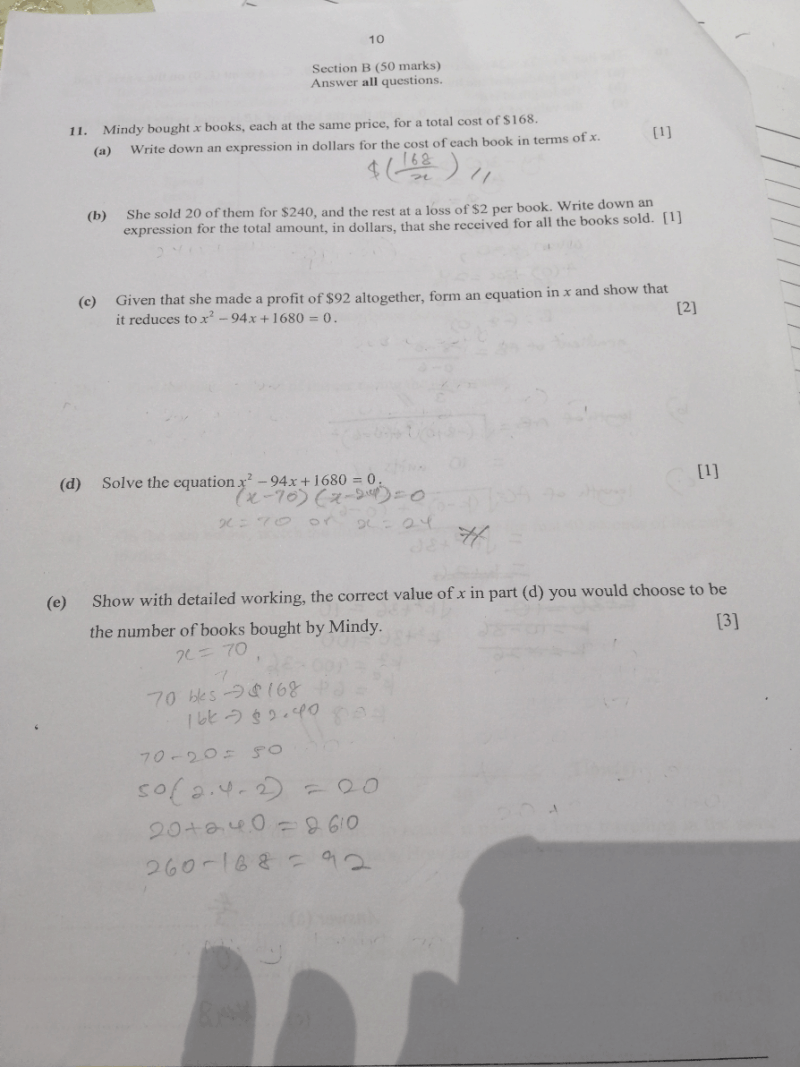# QuestionHow to solve part E). Not sure how to explain.

Not exactly sure what the question wants either, especially since both solutions are equally valid. I would simply substitute the solutions as two separate scenarios to show that they are both valid.

Scenario 1: x = 70 | Mindy bought 70 books

Cost price per book: \$168 ÷ 70 = \$2.40
# of books left after selling 20 books: 70 – 20 = 50
Price which the remaining 50 books were sold: \$2.40 – \$2 = \$0.40
Earnings from selling these 50 books: 50 x \$0.40 = \$20

Profit: (\$240 + \$20) – \$168 = \$92

Scenario 2: x = 24 | Mindy bought 24 books

Cost price per book: \$168 ÷ 24 = \$7
# of books left after selling 20 books: 24 – 20 = 4
Price which the remaining 4 books were sold: \$7 – \$2 = \$5
Earnings from selling these 4 books = \$20

Profit: (\$240 + \$20) – \$168 = \$92

Since both solutions are positive and real roots which satisfy the equation, both are equally valid in this context.

0 Replies 0 Likes ✔Accepted Answer